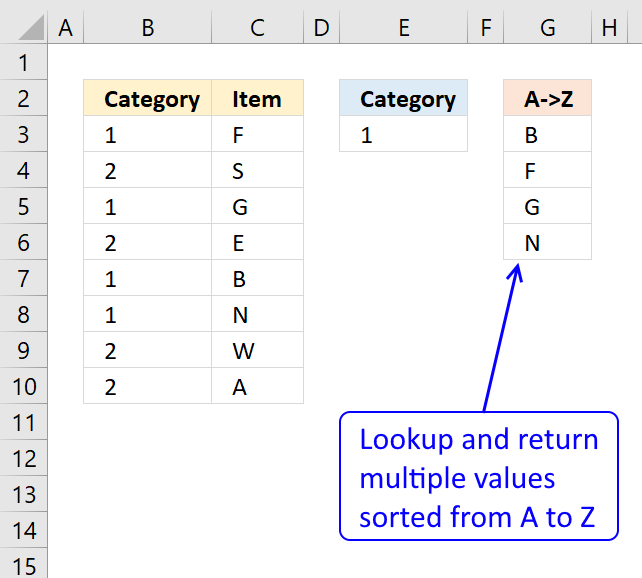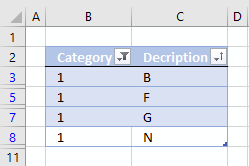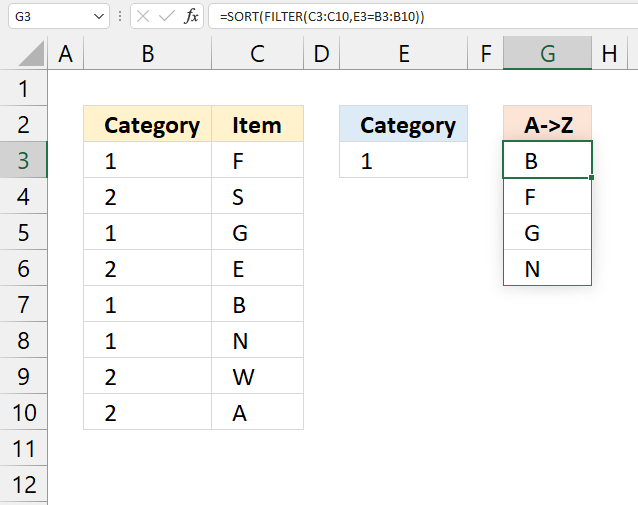Author: Oscar Cronquist Article last updated on February 03, 2023This article demonstrates how to extract multiple values based on a search value and display them sorted from A to Z.

The array formula in column G filters values in column C using a condition in cell E3, comparing it with values in adjacent column B. The filtered values are then sorted from A to Z.

It is possible to build a formula around the VLOOKUP function but it would be big, the following formula is smaller and easier to understand.

## 1. Extract multiple values based on a search value sorted from A to ZThis example demonstrates a formula that works in most Excel versions. The formula in cell G3 extracts values from column C if the corresponding value on the same row matches the search value specified in cell E3.

The result is sorted from A to Z and displayed in cell G3 and cells below as far as needed.

Array formula in cell G3:

=INDEX(\$C\$3:\$C\$10, MATCH(SMALL(IF(\$E\$3=\$B\$3:\$B\$10, COUNTIF(\$C\$3:\$C\$10, "<"&\$C\$3:\$C\$10), ""),ROWS(\$A\$1:A1)), COUNTIF(\$C\$3:\$C\$10,"<"&\$C\$3:\$C\$10), 0))

### 1.1 Watch a video where I explain the formula

Recommended article

Recommended articles

Unique distinct list sorted alphabetically based on a condition
This article demonstrates how to extract unique distinct values based on a condition and also sorted from A to z. […]

### 1.2 How to enter an array formula

1. Double press with the left mouse button on cell G3.
2. Copy and paste the above formula to cell G3.
3. Press and hold CTRL + SHIFT simultaneously.
4. Press Enter once.
5. Release all keys.

Take a look at the formula bar and you will see that the formula now has a beginning and ending curly bracket.

Don't enter these characters yourself, they appear automatically. Example, {=array_formula}The image above demonstrates the location of the formula bar.

Recommended article

Recommended articles

A beginners guide to Excel array formulas
Array formulas allows you to do advanced calculations not possible with regular formulas.

### 1.3 Explaining formula in cell G3

#### Step 1 - Sort values in column C

COUNTIF(\$C\$3:\$C\$10, "<"&\$C\$3:\$C\$10)

becomes

COUNTIF({"F"; "S"; "G"; "E"; "B"; "N"; "W"; "A"},{"<F"; "<S"; "<G"; "<E"; "<B"; "<N"; "<W"; "<A"})

and returns

{3;6;4;2;1;5;7;0}

Recommended article

Recommended articles

How to use the COUNTIF function
Counts the number of cells that meet a specific condition.

#### Step 2 - Extract sort rank numbers for chosen category

IF(\$E\$3=\$B\$3:\$B\$10, COUNTIF(\$C\$3:\$C\$10, "<"&\$C\$3:\$C\$10), "")

becomes

IF(\$E\$3=\$B\$3:\$B\$10, {3;6;4;2;1;5;7;0}, "")

becomes

IF(\$E\$3=\$B\$3:\$B\$10, {3;6;4;2;1;5;7;0}, "")

becomes

IF(1={1;2;1;2;1;1;2;2},{3;6;4;2;1;5;7;0},"")

becomes

IF({TRUE;FALSE;TRUE;FALSE;TRUE;TRUE;FALSE;FALSE},{3;6;4;2;1;5;7;0},"")

and returns

{3;"";4;"";1;5;"";""}

Recommended article

Recommended articles

How to use the IF function
Checks if a logical expression is met. Returns a specific value if TRUE and another specific value if FALSE.

#### Step 3 - Find k-th smallest value in array

SMALL(IF(\$E\$3=\$B\$3:\$B\$10,COUNTIF(\$C\$3:\$C\$10,"<"&\$C\$3:\$C\$10),""),ROWS(\$A\$1:A1))

becomes

SMALL({3;"";4;"";1;5;"";""},ROWS(\$A\$1:A1))

becomes

SMALL({3;"";4;"";1;5;"";""},1)

and returns 1.

Recommended article

Recommended articles

How to use the SMALL function
The SMALL function lets you extract a number in a cell range based on how small it is compared to the other numbers in the group.

#### Step 4 - Match sort rank to find relative position

MATCH(SMALL(IF(\$E\$3=\$B\$3:\$B\$10, COUNTIF(\$C\$3:\$C\$10, "<"&\$C\$3:\$C\$10), ""),ROWS(\$A\$1:A1)), COUNTIF(\$C\$3:\$C\$10,"<"&\$C\$3:\$C\$10), 0)

becomes

MATCH(1, {3;6;4;2;1;5;7;0}, 0)

and returns 5.

Recommended article

Recommended articles

How to use the MATCH function
Identify the position of a value in an array.

#### Step 5 - Return values

INDEX(\$C\$3:\$C\$10,MATCH(SMALL(IF(\$E\$3=\$B\$3:\$B\$10,COUNTIF(\$C\$3:\$C\$10,"<"&\$C\$3:\$C\$10),""),ROWS(\$A\$1:A1)),COUNTIF(\$C\$3:\$C\$10,"<"&\$C\$3:\$C\$10),0))

becomes

INDEX(\$C\$3:\$C\$10,5)

becomes

INDEX({"F";"S";"G";"E";"B";"N";"W";"A"},5)

and returns B in cell G3.

Recommended article

Recommended articles

How to use the INDEX function
Gets a value in a specific cell range based on a row and column number.

Tip! You can easily filter values if you convert your data to an excel table and then sort them:Recommended articles

How to use Excel Tables
An Excel table allows you to easily sort, filter and sum values in a data set where values are related.

## 2. Extract multiple values based on a search value sorted from A to Z (Excel 365)This example demonstrates a regular formula that works only in Excel 365. The formula is a dynamic array formula in cell G3, it returns multiple values that spills to cells below automatically.

It contains two functions, the FILTER function and the SORT function. It extracts values from column C if the value on the same row in column B matches the search value specified in cell E3.

Formula in cell G3:

=SORT(FILTER(C3:C10,E3=B3:B10))

### 2.1 Explaining formula

#### Step 1 - Filter values based on a condition

The FILTER function extracts values/rows based on a condition or criteria.

FILTER(arrayinclude, [if_empty])

FILTER(C3:C10,E3=B3:B10)

becomes

FILTER({"F"; "S"; "G"; "E"; "B"; "N"; "W"; "A"}, 1={1; 2; 1; 2; 1; 1; 2; 2})

becomes

FILTER({"F"; "S"; "G"; "E"; "B"; "N"; "W"; "A"}, {TRUE; FALSE; TRUE; FALSE; TRUE; TRUE; FALSE; FALSE})

and returns {"F"; "G"; "B"; "N"}.

#### Step 2 - Sort result

The SORT function lets you sort values from a cell range or array.

SORT(array, [sort_index], [sort_order], [by_col])

SORT(FILTER(C3:C10,E3=B3:B10))

becomes

SORT({"F"; "G"; "B"; "N"})

and returns {"B"; "F"; "G"; "N"}.